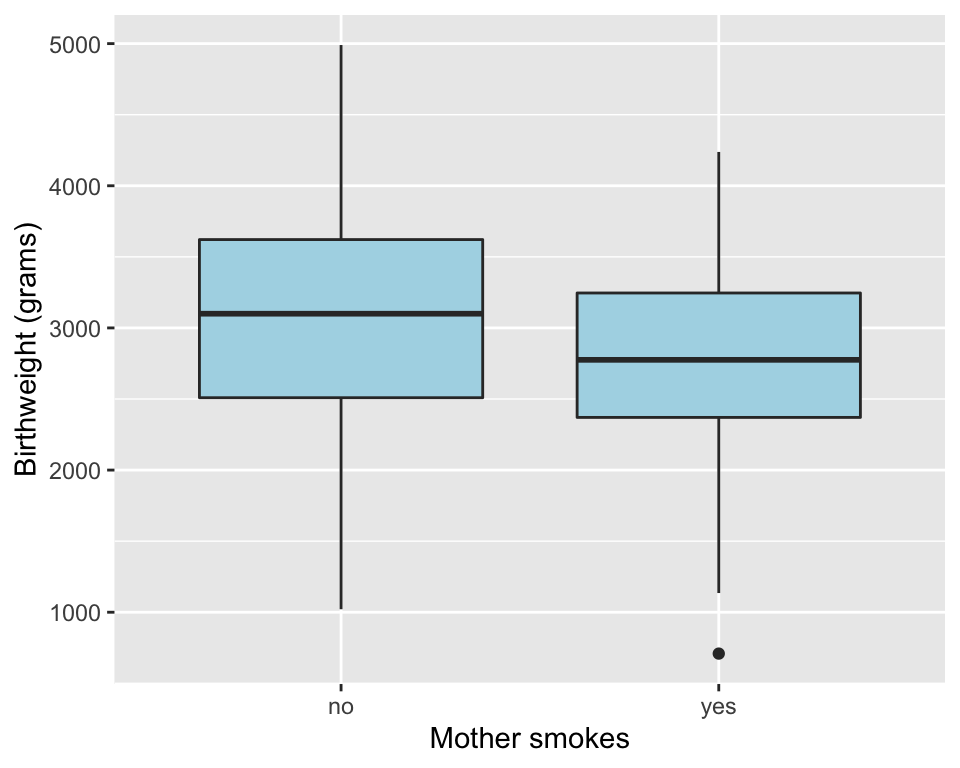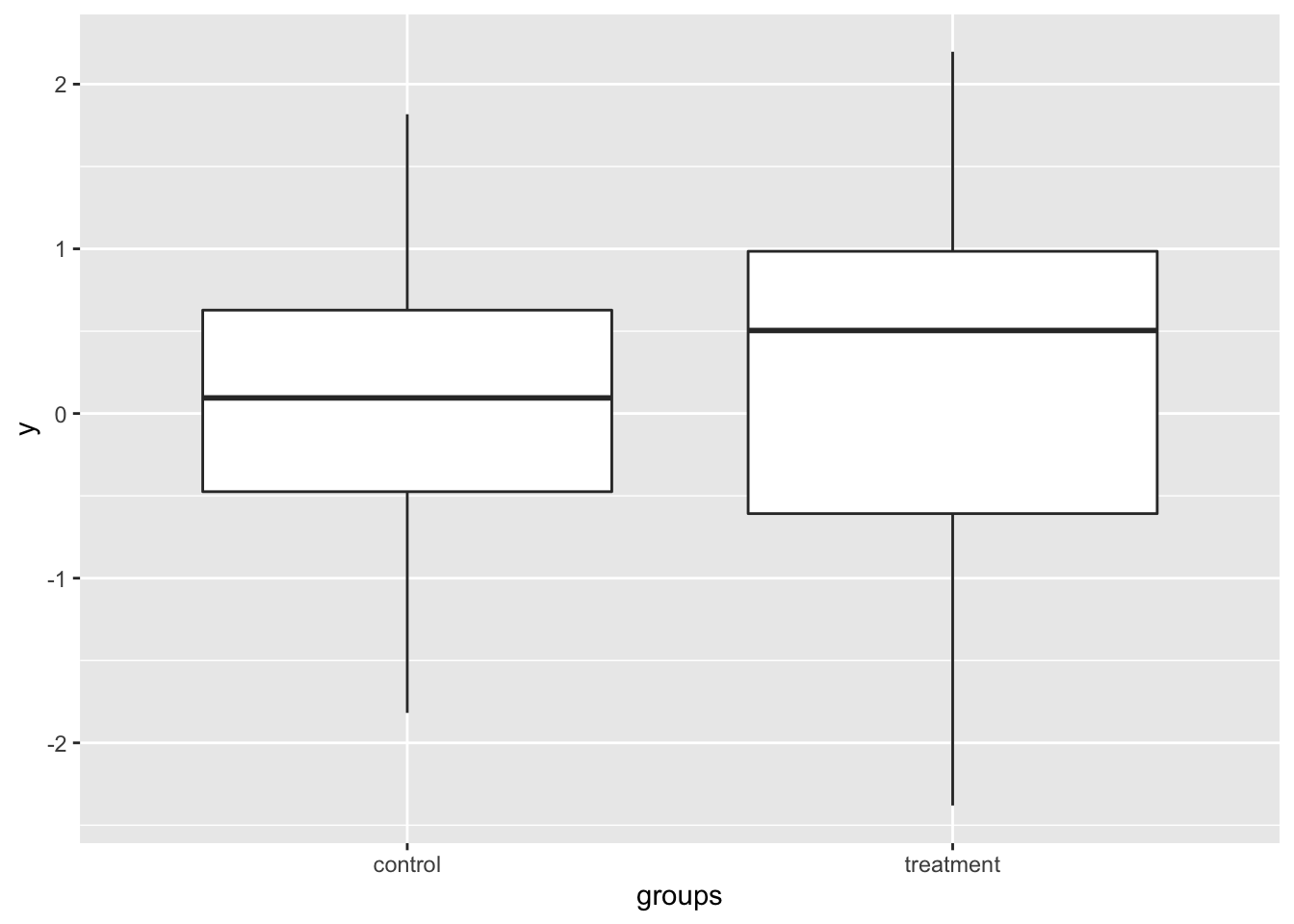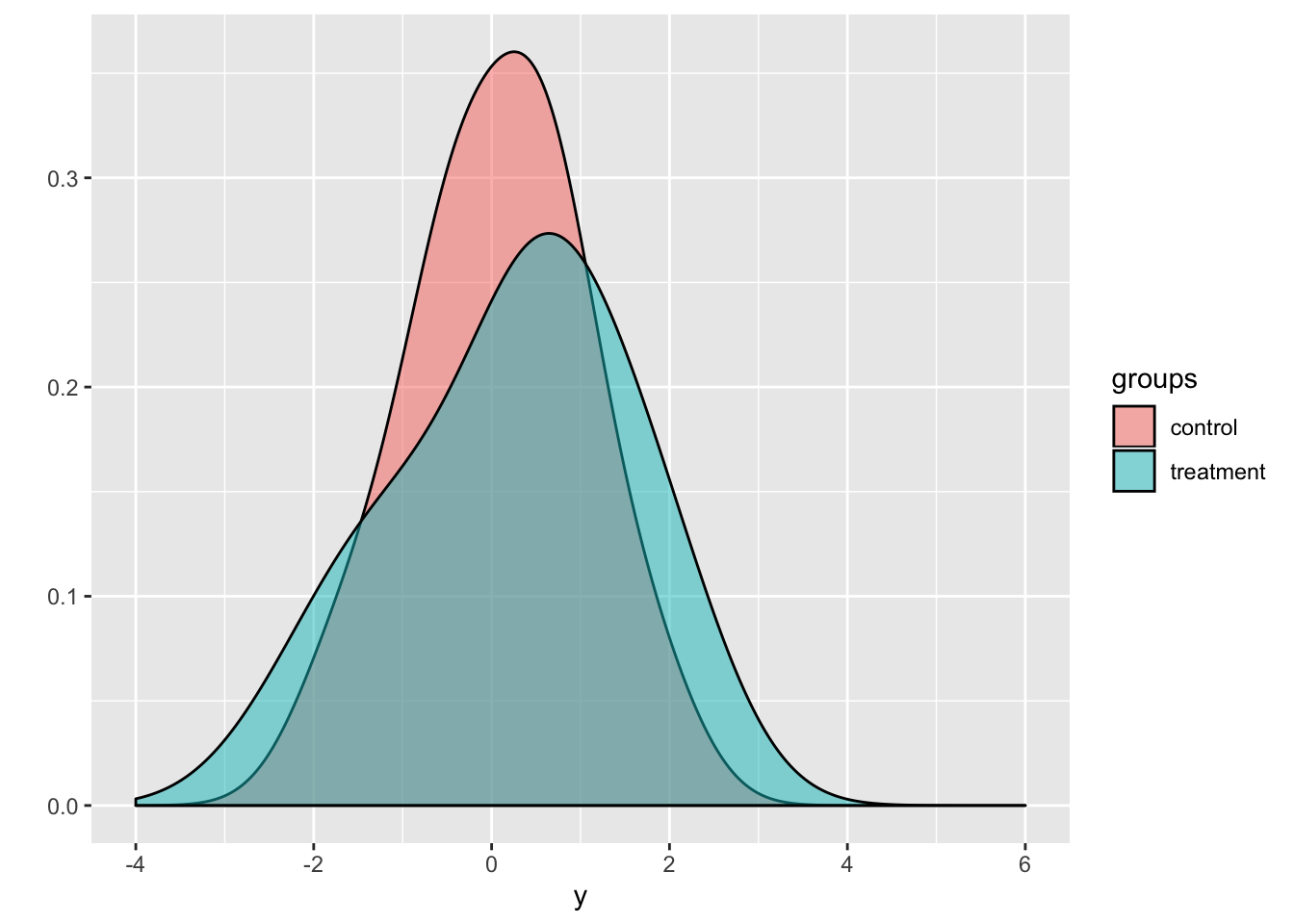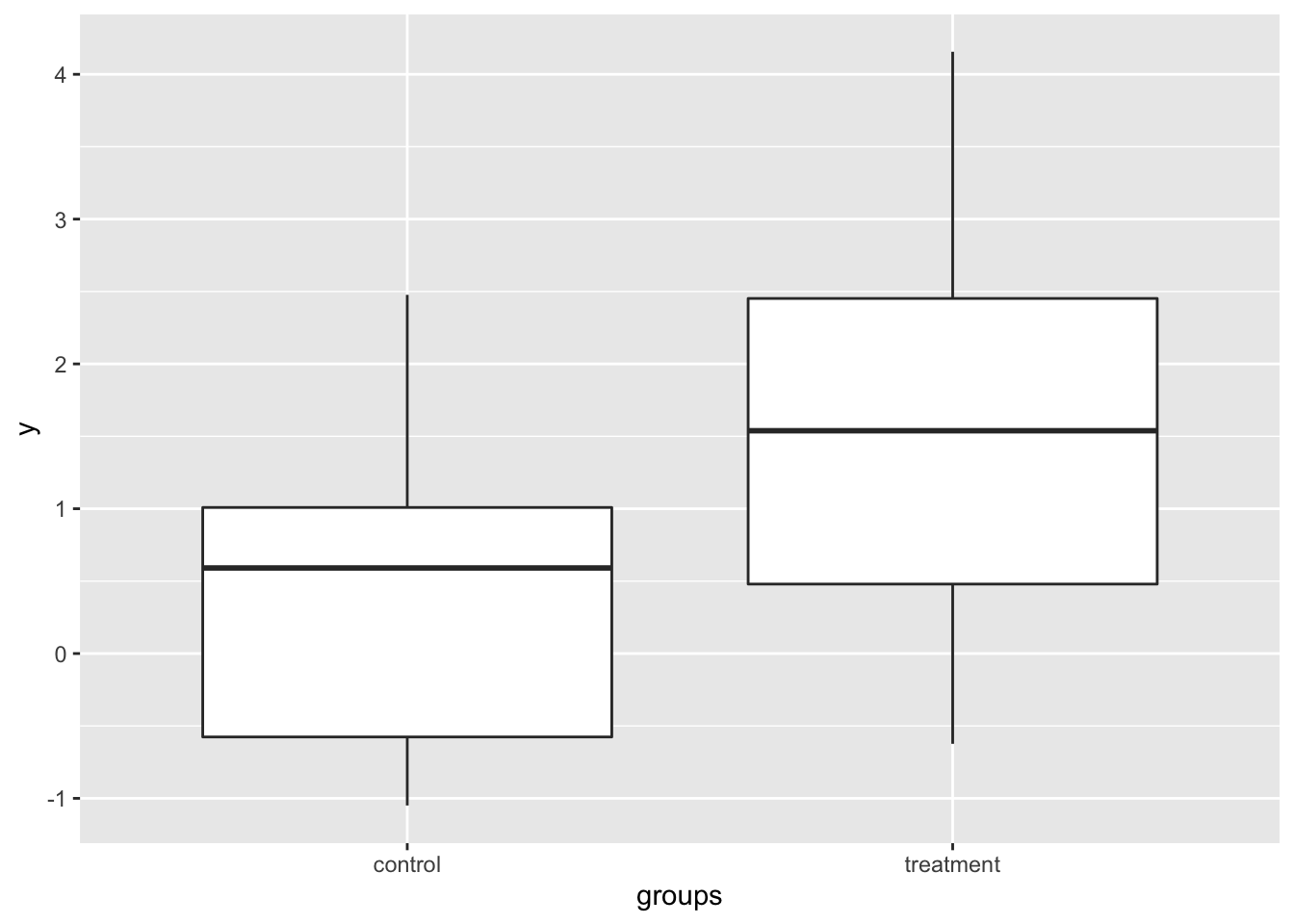##Agenda

• Testing differences in mean between two groups

• QQ plots

• Tests for 2 x 2 tables, j x k tables

• Plotting confidence intervals

``library(tidyverse)``
``## ── Attaching packages ────────────────────────── tidyverse 1.2.1 ──``
``````## ✔ ggplot2 3.2.1     ✔ purrr   0.3.3
## ✔ tibble  2.1.3     ✔ dplyr   0.8.3
## ✔ tidyr   1.0.0     ✔ stringr 1.4.0
## ✔ readr   1.3.1     ✔ forcats 0.4.0``````
``````## ── Conflicts ───────────────────────────── tidyverse_conflicts() ──

## Exploring the birthwt data

We’ll begin by doing all the same data processing as in previous lectures

``````# Load data from MASS into a tibble
birthwt <- as_tibble(MASS::birthwt)

# Rename variables
birthwt <- birthwt %>%
rename(birthwt.below.2500 = low,
mother.age = age,
mother.weight = lwt,
mother.smokes = smoke,
previous.prem.labor = ptl,
hypertension = ht,
uterine.irr = ui,
physician.visits = ftv,
birthwt.grams = bwt)

# Change factor level names
birthwt <- birthwt %>%
mutate(race = recode_factor(race, `1` = "white", `2` = "black", `3` = "other")) %>%
mutate_at(c("mother.smokes", "hypertension", "uterine.irr", "birthwt.below.2500"),
~ recode_factor(.x, `0` = "no", `1` = "yes"))``````

Over the past two lectures we created various tables and graphics to help us better understand the data. Our focus for today is to run hypothesis tests to assess whether the trends we observed last time are statistically significant.

One of the main reasons we want to understand hypothesis testing is that it is important for our tables and figures to convey statistical uncertainty in any cases where it is non-negligible, and where failing to account for it may produce misleading conclusions.

### Testing differences in means

One of the most common statistical tasks is to compare an outcome between two groups. The example here looks at comparing birth weight between smoking and non-smoking mothers.

To start, it always helps to plot things

``````# Create boxplot showing how birthwt.grams varies between
# smoking status
qplot(x = mother.smokes, y = birthwt.grams,
geom = "boxplot", data = birthwt,
xlab = "Mother smokes",
ylab = "Birthweight (grams)",
fill = I("lightblue"))``````This plot suggests that smoking is associated with lower birth weight.

How can we assess whether this difference is statistically significant?

Let’s compute a summary table

``````# Notice the consistent use of round() to ensure that our summaries
# do not have too many decimal values
birthwt %>%
group_by(mother.smokes) %>%
summarize(mean.birthwt = round(mean(birthwt.grams), 0),
sd.birthwt = round(sd(birthwt.grams), 0))``````
``````## # A tibble: 2 x 3
##   mother.smokes mean.birthwt sd.birthwt
##   <fct>                <dbl>      <dbl>
## 1 no                    3056        753
## 2 yes                   2772        660``````

The standard deviation is good to have, but to assess statistical significance we really want to have the standard error (which the standard deviation adjusted by the group size).

``````birthwt %>%
group_by(mother.smokes) %>%
summarize(num.obs = n(),
mean.birthwt = round(mean(birthwt.grams), 0),
sd.birthwt = round(sd(birthwt.grams), 0),
se.birthwt = round(sd(birthwt.grams) / sqrt(num.obs), 0))``````
``````## # A tibble: 2 x 5
##   mother.smokes num.obs mean.birthwt sd.birthwt se.birthwt
##   <fct>           <int>        <dbl>      <dbl>      <dbl>
## 1 no                115         3056        753         70
## 2 yes                74         2772        660         77``````

#### t-test via t.test()

This difference is looking quite significant. To run a two-sample t-test, we can simple use the `t.test()` function.

``````birthwt.t.test <- t.test(birthwt.grams ~ mother.smokes, data = birthwt)
birthwt.t.test``````
``````##
##  Welch Two Sample t-test
##
## data:  birthwt.grams by mother.smokes
## t = 2.7299, df = 170.1, p-value = 0.007003
## alternative hypothesis: true difference in means is not equal to 0
## 95 percent confidence interval:
##   78.57486 488.97860
## sample estimates:
##  mean in group no mean in group yes
##          3055.696          2771.919``````

We see from this output that the difference is highly significant. The `t.test()` function also outputs a confidence interval for us.

Notice that the function returns a lot of information, and we can access this information element by element

``names(birthwt.t.test)``
``````##   "statistic"   "parameter"   "p.value"     "conf.int"    "estimate"
##   "null.value"  "stderr"      "alternative" "method"      "data.name"``````
``birthwt.t.test\$p.value   # p-value``
``##  0.007002548``
``birthwt.t.test\$estimate  # group means``
``````##  mean in group no mean in group yes
##          3055.696          2771.919``````
``birthwt.t.test\$conf.int  # confidence interval for difference``
``````##   78.57486 488.97860
## attr(,"conf.level")
##  0.95``````
``attr(birthwt.t.test\$conf.int, "conf.level")  # confidence level``
``##  0.95``

The ability to pull specific information from the output of the hypothesis test allows you to report your results using inline code chunks. That is, you don’t have to hardcode estimates, p-values, confidence intervals, etc.

``````# Calculate difference in means between smoking and nonsmoking groups
birthwt.t.test\$estimate``````
``````##  mean in group no mean in group yes
##          3055.696          2771.919``````
``````birthwt.smoke.diff <- round(birthwt.t.test\$estimate - birthwt.t.test\$estimate, 1)

# Confidence level as a %
conf.level <- attr(birthwt.t.test\$conf.int, "conf.level") * 100``````

Example: Here’s what happens when we knit the following paragraph.

``Our study finds that birth weights are on average `r birthwt.smoke.diff`g higher in the non-smoking group compared to the smoking group (t-statistic `r round(birthwt.t.test\$statistic,2)`, p=`r round(birthwt.t.test\$p.value, 3)`, `r conf.level`% CI [`r round(birthwt.t.test\$conf.int,1)`]g)``

Output: Our study finds that birth weights are on average 283.8g higher in the non-smoking group compared to the smoking group (t-statistic 2.73, p=0.007, 95% CI [78.6, 489]g)

One other thing to know is that `t.test()` accepts input in multiple forms. I like using the formula form whenever it’s available, as I find it to be more easily interpretable. Here’s another way of specifying the same information.

``````with(birthwt, t.test(x=birthwt.grams[mother.smokes=="no"],
y=birthwt.grams[mother.smokes=="yes"]))``````
``````##
##  Welch Two Sample t-test
##
## data:  birthwt.grams[mother.smokes == "no"] and birthwt.grams[mother.smokes == "yes"]
## t = 2.7299, df = 170.1, p-value = 0.007003
## alternative hypothesis: true difference in means is not equal to 0
## 95 percent confidence interval:
##   78.57486 488.97860
## sample estimates:
## mean of x mean of y
##  3055.696  2771.919``````

Specifying `x` and `y` arguments to the `t.test` function runs a t-test to check whether `x` and `y` have the same mean.

#### What is statistical significance testing doing?

Here’s a little simulation where we have two groups, a treatment groups and a control group. We’re going to simulate observations from both groups. We’ll run the simulation two ways.

• First simulation (Null case): the treatment has no effect
• Second simulation (Non-null case): the treatment on average increases outcome
``````set.seed(12345)
# Function to generate data
generateSimulationData <- function(n1, n2, mean.shift = 0) {
y <- rnorm(n1 + n2) + c(rep(0, n1), rep(mean.shift, n2))
groups <- c(rep("control", n1), rep("treatment", n2))
data.frame(y = y, groups = groups)
}``````

Let’s look at a single realization in the null setting.

``````n1 = 30
n2 = 40
# Observation, null case
obs.data <- generateSimulationData(n1 = n1, n2 = n2)
obs.data``````
``````##              y    groups
## 1   0.58552882   control
## 2   0.70946602   control
## 3  -0.10930331   control
## 4  -0.45349717   control
## 5   0.60588746   control
## 6  -1.81795597   control
## 7   0.63009855   control
## 8  -0.27618411   control
## 9  -0.28415974   control
## 10 -0.91932200   control
## 11 -0.11624781   control
## 12  1.81731204   control
## 13  0.37062786   control
## 14  0.52021646   control
## 15 -0.75053199   control
## 16  0.81689984   control
## 17 -0.88635752   control
## 18 -0.33157759   control
## 19  1.12071265   control
## 20  0.29872370   control
## 21  0.77962192   control
## 22  1.45578508   control
## 23 -0.64432843   control
## 24 -1.55313741   control
## 25 -1.59770952   control
## 26  1.80509752   control
## 27 -0.48164736   control
## 28  0.62037980   control
## 29  0.61212349   control
## 30 -0.16231098   control
## 31  0.81187318 treatment
## 32  2.19683355 treatment
## 33  2.04919034 treatment
## 34  1.63244564 treatment
## 35  0.25427119 treatment
## 36  0.49118828 treatment
## 37 -0.32408658 treatment
## 38 -1.66205024 treatment
## 39  1.76773385 treatment
## 40  0.02580105 treatment
## 41  1.12851083 treatment
## 42 -2.38035806 treatment
## 43 -1.06026555 treatment
## 44  0.93714054 treatment
## 45  0.85445172 treatment
## 46  1.46072940 treatment
## 47 -1.41309878 treatment
## 48  0.56740325 treatment
## 49  0.58318765 treatment
## 50 -1.30679883 treatment
## 51 -0.54038607 treatment
## 52  1.94769266 treatment
## 53  0.05359027 treatment
## 54  0.35166284 treatment
## 55 -0.67097654 treatment
## 56  0.27795369 treatment
## 57  0.69117127 treatment
## 58  0.82379533 treatment
## 59  2.14506502 treatment
## 60 -2.34694398 treatment
## 61  0.14959198 treatment
## 62 -1.34253148 treatment
## 63  0.55330308 treatment
## 64  1.58996284 treatment
## 65 -0.58687959 treatment
## 66 -1.83237731 treatment
## 67  0.88813943 treatment
## 68  1.59348847 treatment
## 69  0.51685467 treatment
## 70 -1.29567168 treatment``````
``````# Box plots
qplot(x = groups, y = y, data = obs.data, geom = "boxplot")````````````# Density plots
qplot(fill = groups, x = y, data = obs.data, geom = "density",
alpha = I(0.5),
xlim = c(-4, 6))````````````# t-test
t.test(y ~ groups, data = obs.data)``````
``````##
##  Welch Two Sample t-test
##
## data:  y by groups
## t = -0.61095, df = 67.998, p-value = 0.5433
## alternative hypothesis: true difference in means is not equal to 0
## 95 percent confidence interval:
##  -0.6856053  0.3641889
## sample estimates:
##   mean in group control mean in group treatment
##              0.07880701              0.23951518``````

And here’s what happens in a random realization in the non-null setting.

``````# Non-null case, very strong treatment effect
# Observation, null case
obs.data <- generateSimulationData(n1 = n1, n2 = n2, mean.shift = 1.5)

# Box plots
qplot(x = groups, y = y, data = obs.data, geom = "boxplot")````````````# Density plots
qplot(fill = groups, x = y, data = obs.data, geom = "density",
alpha = I(0.5),